• Slides: 11Properties ofof Quadratic Functions in Properties Quadratic 5 -1 Standard Form Functions in Standard Form SWBAT Define, identify, and graph quadratic functions. Identify andidentify, use and maximums and minimums ofuse SWBAT: Define, identify, and graph quadratic functions. Identify and use maximums minimums of quadratic functions to solve problems. Holt. Mc. Dougal Algebraand 2 Algebra Holt 25 -1 Properties of Quadratic Functions in Standard Form Vocabulary • Quadratic Function: – An equation with a degree of 2. • Standard Form: – y = ax 2 + bx + c • Parabola: – A quadratic graph that has a U shape • Vertex: – The lowest or highest point of the graph • Axis of Symmetry: – The line passing through the vertex that divides thee parabola in half SWBAT: Define, identify, and graph quadratic functions. Identify and use maximums and minimums of quadratic functions to solve problems. Holt Mc. Dougal Algebra 25 -1 Properties of Quadratic Functions in Standard Form SWBAT: Define, identify, and graph quadratic functions. Identify and use maximums and minimums of quadratic functions to solve problems. Holt Mc. Dougal Algebra 25 -1 Properties of Quadratic Functions in Standard Form This shows that parabolas are symmetric curves. The axis of symmetry is the line through the vertex of a parabola that divides the parabola into two congruent halves. SWBAT: Define, identify, and graph quadratic functions. Identify and use maximums and minimums of quadratic functions to solve problems. Holt Mc. Dougal Algebra 2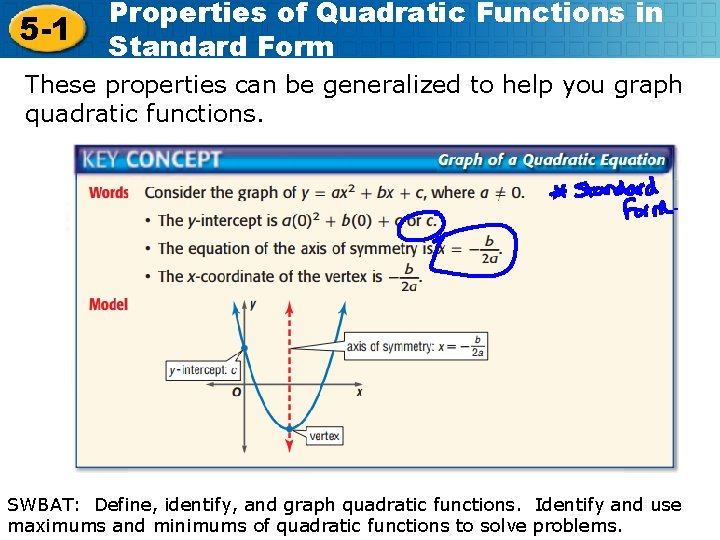5 -1 Properties of Quadratic Functions in Standard Form These properties can be generalized to help you graph quadratic functions. SWBAT: Define, identify, and graph quadratic functions. Identify and use maximums and minimums of quadratic functions to solve problems. Holt Mc. Dougal Algebra 2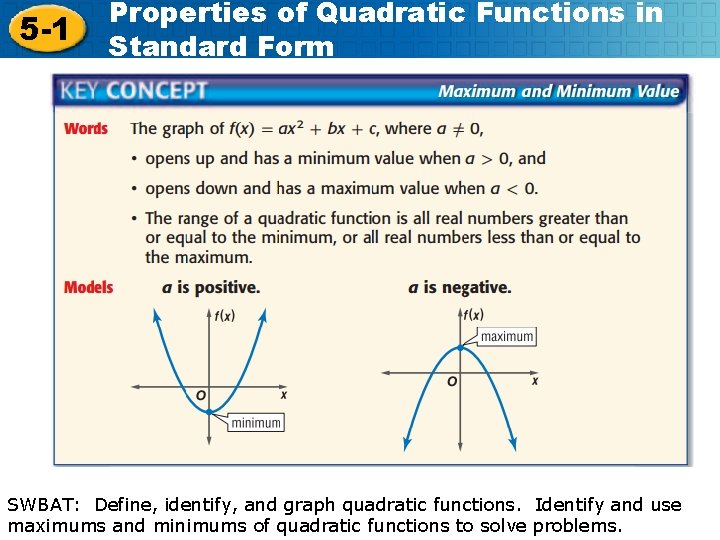5 -1 Properties of Quadratic Functions in Standard Form SWBAT: Define, identify, and graph quadratic functions. Identify and use maximums and minimums of quadratic functions to solve problems. Holt Mc. Dougal Algebra 2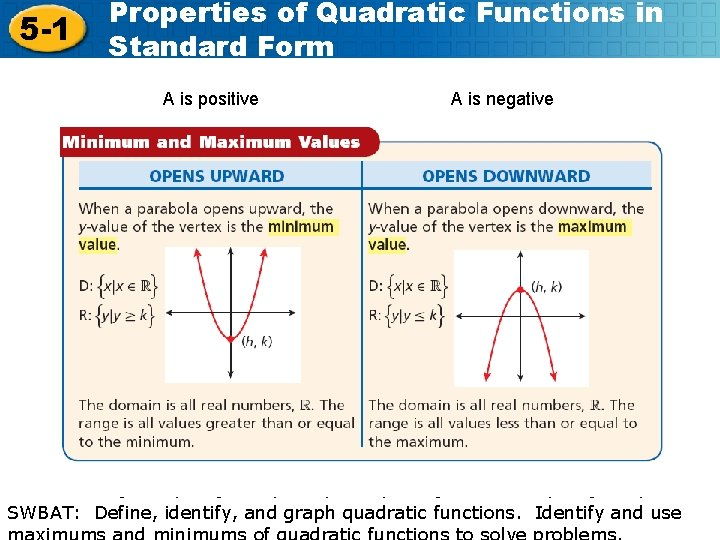5 -1 Properties of Quadratic Functions in Standard Form A is positive A is negative SWBAT: Define, identify, and graph quadratic functions. Identify and use maximums and minimums of quadratic functions to solve problems. Holt Mc. Dougal Algebra 2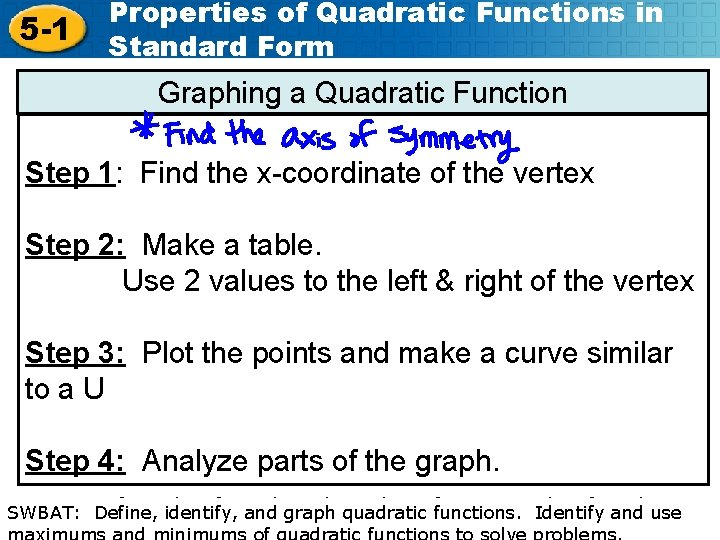5 -1 Properties of Quadratic Functions in Standard Form Graphing a Quadratic Function Step 1: Find the x-coordinate of the vertex Step 2: Make a table. Use 2 values to the left & right of the vertex Step 3: Plot the points and make a curve similar to a U Step 4: Analyze parts of the graph. SWBAT: Define, identify, and graph quadratic functions. Identify and use maximums and minimums of quadratic functions to solve problems. Holt Mc. Dougal Algebra 2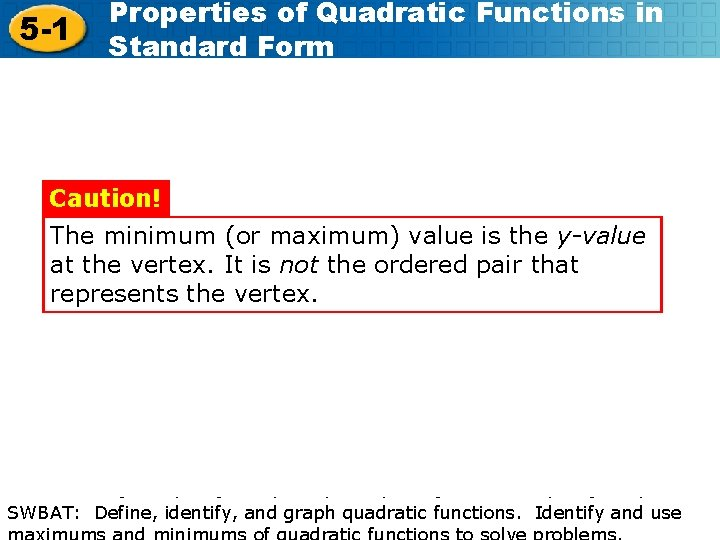5 -1 Properties of Quadratic Functions in Standard Form Caution! The minimum (or maximum) value is the y-value at the vertex. It is not the ordered pair that represents the vertex. SWBAT: Define, identify, and graph quadratic functions. Identify and use maximums and minimums of quadratic functions to solve problems. Holt Mc. Dougal Algebra 2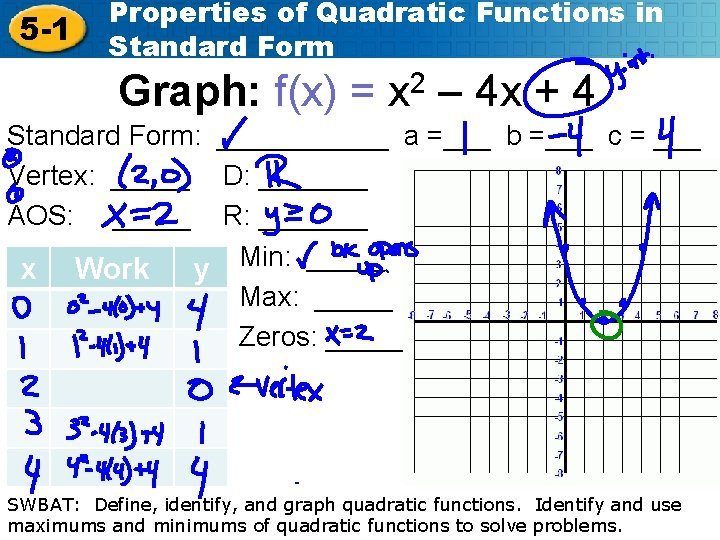5 -1 Properties of Quadratic Functions in Standard Form Graph: f(x) = x 2 – 4 x + 4 Standard Form: ______ a =___ b =___ c = ___ Vertex: _____ D: _______ AOS: _____ R: _______ x Work y Min: _____ Max: _____ Zeros: _____ SWBAT: Define, identify, and graph quadratic functions. Identify and use maximums and minimums of quadratic functions to solve problems. Holt Mc. Dougal Algebra 2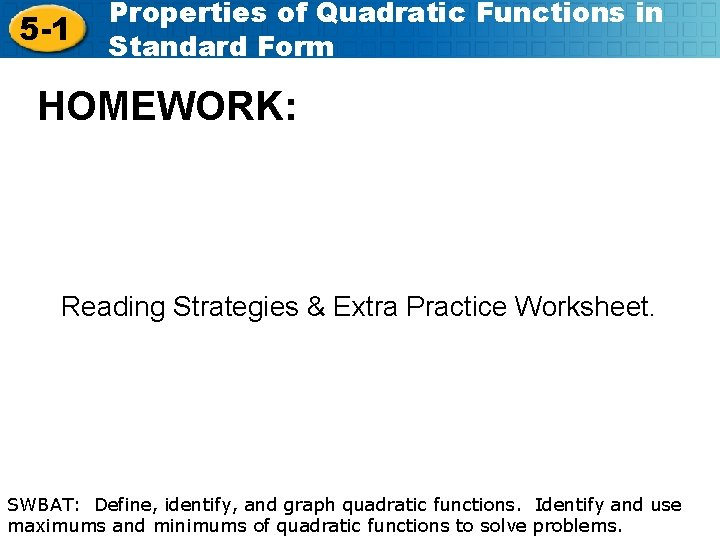5 -1 Properties of Quadratic Functions in Standard Form HOMEWORK: Reading Strategies & Extra Practice Worksheet. SWBAT: Define, identify, and graph quadratic functions. Identify and use maximums and minimums of quadratic functions to solve problems. Holt Mc. Dougal Algebra 2